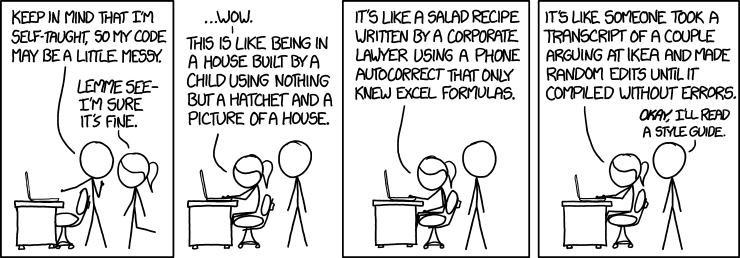1. ## Code QualityTagged as : humor xkcd programming
2. ## Fizz Buzz

Problem:

Write a program that prints the numbers from 1 to 100. But for multiples of three print “Fizz” instead of the number and for the multiples of five print “Buzz”. For numbers which are multiples of both three and five print “FizzBuzz”.

`f = lambda x: 'FizzBuzz' if not (x …`
Tagged as : python programming
3. ## Using timezone with datetime in python

```>>> import pytz
>>> import datetime
>>> tz = pytz.timezone('Asia/Calcutta')
>>> tz
<DstTzInfo 'Asia/Calcutta' HMT+5:53:00 STD>
>>> t = tz.localize(datetime.datetime(2014, 4, 5, 6, 7, 8, 900))
>>> t
datetime.datetime(2014, 4, 5, 6, 7, 8, 900, tzinfo=<DstTzInfo 'Asia/Calcutta' IST+5:30:00 STD>)
>>> t …```
4. ## Creating class in python without using class keyword

```add2 = lambda x,y: x+y
sub2 = lambda x,y: x-y
mul2 = lambda x,y: x*y
# Second parameter is tuple, used for inheriting classes
# Third parameter is dict, used for defining variables and functions
myclass = type('myclass', (), {'var1': 10, 'add': add2, 'sub': sub2, 'mul': mul2})
myinstance = myclass()
print …```
Tagged as : python programming
5. ## Reversing the binary of the given number in python

```>>> a=int(input('Enter base 10 natural number: '))
Enter base 10 natural number: 42
>>> b = bin(a)
>>> a = int(b[:2]+b[2:][::-1], 2)
>>> a
21
```

Page 1 / 1